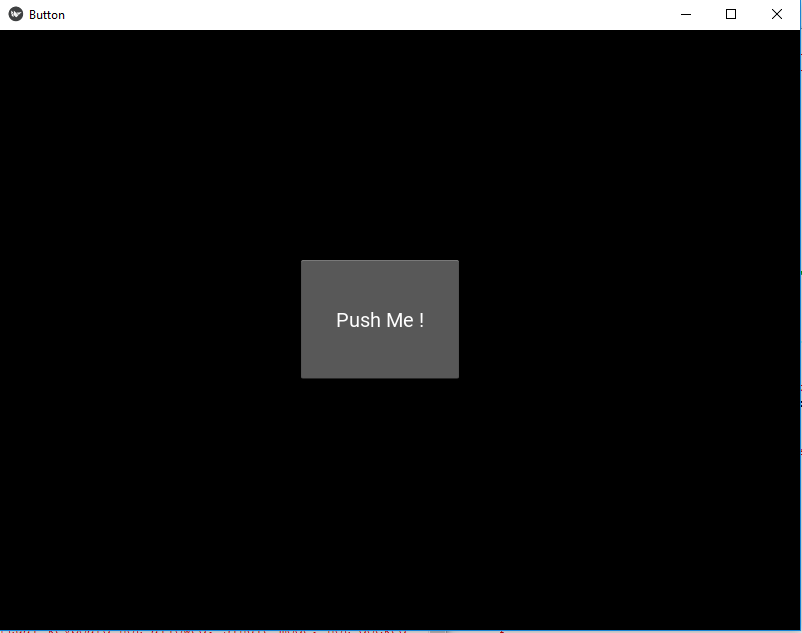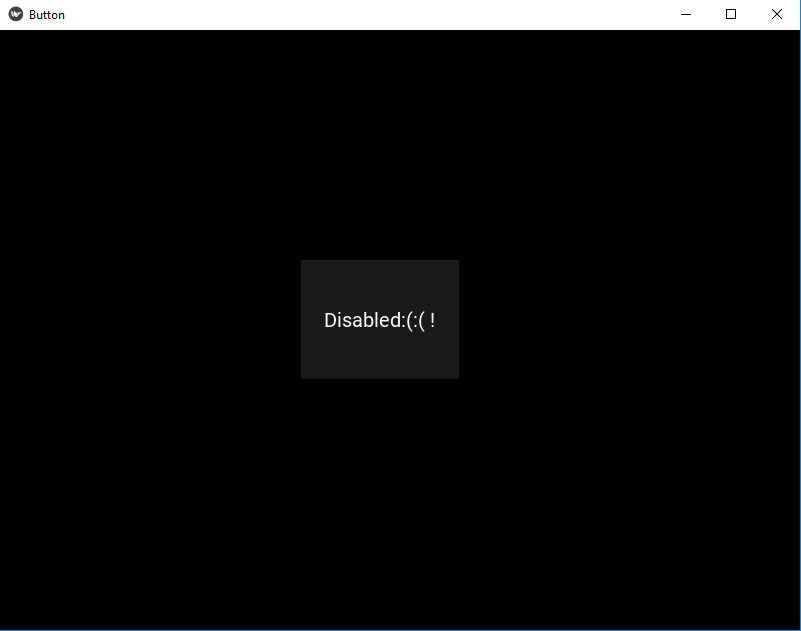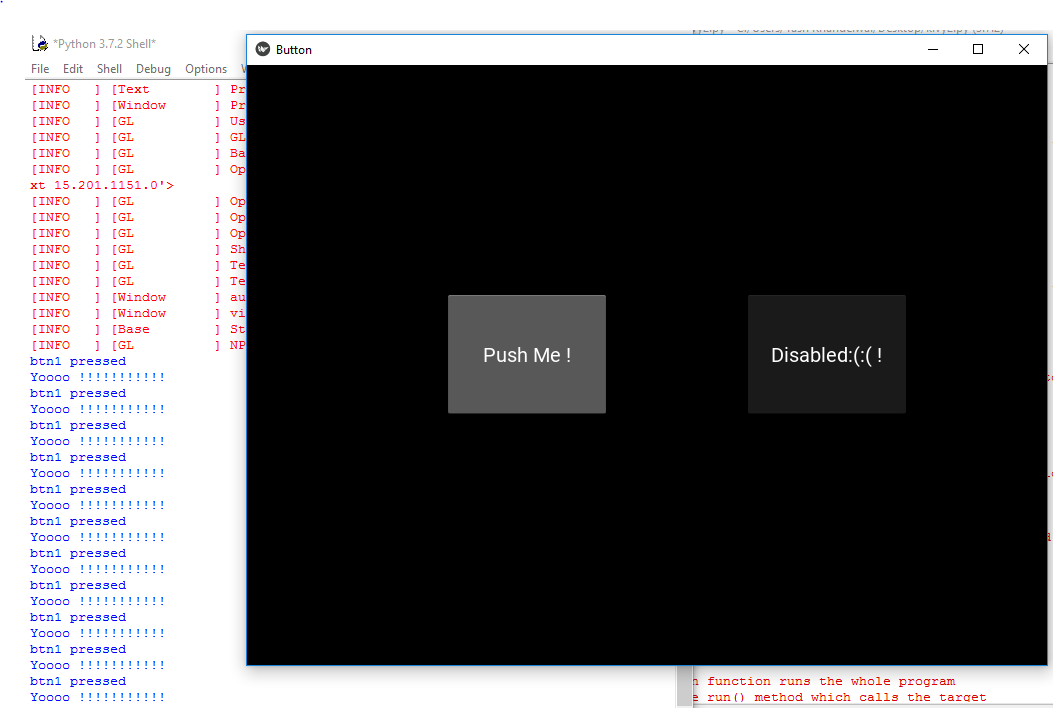GeeksforGeeks App
Open AppBrowser
Continue

In this article, we will learn how to disable a button in kivy, there are some places where we need to disable the buttons So in this article you will learn how to do that.

Kivy Tutorial – Learn Kivy with Examples.

The Button is a Label with associated actions that are triggered when the button is pressed (or released after a click/touch). We can add functions behind the button and style the button.But to disable the button we have a property name :

`disabled that must be true`

this property will help in disabling the button i.e. button will be there but is of no use as it is disabled no functionality of button will work.

Note: disabled property was introduced in version 1.8.0. If you want to use it you need to actualize your framework.

Basic Approach to follow while creating and disabling button :

-> import kivy
-> import kivy App
-> import button
-> set minimum version(optional)
-> Extend the class
-> Add and return a button
-> Add disabled = true to disable button
-> Run an instance of the class

Firstly, let’s see how to create a fully working button and then see how to disable it and its functionality.

Code #1: How to create fully working button

 `def` `build(``self``):``    ``# use a (r, g, b, a) tuple``    ``btn ``=` `Button(text ``=``"Push Me !"``,``                 ``font_size ``=``"20sp"``,``                 ``background_color ``=``(``1``, ``1``, ``1``, ``1``),``                 ``color ``=``(``1``, ``1``, ``1``, ``1``),``                 ``size ``=``(``32``, ``32``),``                 ``size_hint ``=``(.``2``, .``2``),``                 ``pos ``=``(``300``, ``250``))`` ` `    ``return` `btn`

Output:Code #2: How to disable the button

 `def` `build(``self``):``        ``# use a (r, g, b, a) tuple``        ``btn ``=` `Button(text ``=``"Push Me !"``,``                     ``font_size ``=``"20sp"``,``                     ``background_color ``=``(``1``, ``4``, ``6``, ``1``),``                     ``color ``=``(``1``, ``1``, ``1``, ``1``),``                     ``size ``=``(``32``, ``32``),``                     ``size_hint ``=``(.``2``, .``2``),``                     ``pos ``=``(``300``, ``250``),`` ` `                     ``# Disabling the button   ``                     ``disabled ``=` `True`` ` `                     ``)`

Output:Code #3: Both disable and working button together

 `# import kivy module ``import` `kivy ``   ` `# this restrict the kivy version i.e ``# below this kivy version you cannot ``# use the app or software ``kivy.require(``"1.9.1"``) ``   ` `# base Class of your App inherits from the App class. ``# app:always refers to the instance of your application ``from` `kivy.app ``import` `App ``   ` `# creates the button in kivy ``# if not imported shows the error ``from` `kivy.uix.button ``import` `Button `` ` `# This layout allows you to set relative coordinates for children. ``from` `kivy.uix.relativelayout ``import` `RelativeLayout `` ` `# class in which we are creating the button ``class` `ButtonApp(App): ``       ` `    ``def` `build(``self``):`` ` `        ``r1 ``=` `RelativeLayout()`` ` `         ` `        ``# working button``        ``btn1 ``=` `Button(text ``=``"Push Me !"``, ``                   ``font_size ``=``"20sp"``, ``                   ``background_color ``=``(``1``, ``1``, ``1``, ``1``), ``                   ``color ``=``(``1``, ``1``, ``1``, ``1``), ``                   ``size ``=``(``32``, ``32``), ``                   ``size_hint ``=``(.``2``, .``2``), ``                   ``pos ``=``(``200``, ``250``)) `` ` `        ``# disabled button``        ``btn2 ``=` `Button(text ``=``"Disabled:(:( !"``, ``                   ``font_size ``=``"20sp"``, ``                   ``background_color ``=``(``1``, ``1``, ``1``, ``1``), ``                   ``color ``=``(``1``, ``1``, ``1``, ``1``), ``                   ``size ``=``(``32``, ``32``), ``                   ``size_hint ``=``(.``2``, .``2``), ``                   ``pos ``=``(``500``, ``250``),`` ` `                   ``# Add disabled property true to disabled button``                   ``disabled ``=` `True``)`` ` `        ``r1.add_widget(btn1)``        ``r1.add_widget(btn2)``        ` `        ``# bind() use to bind the button to function callback ``        ``btn1.bind(on_press ``=` `self``.callback)``        ``return` `r1 ``   ` `    ``# callback function tells when button pressed ``    ``def` `callback(``self``, event): ``        ``print``(``"button pressed"``) ``        ``print``(``'Yoooo !!!!!!!!!!!'``) ``           ` `   ` `# creating the object root for ButtonApp() class  ``root ``=` `ButtonApp() `` ` `# run function runs the whole program ``# i.e run() method which calls the target ``# function passed to the constructor. ``root.run() `

Output:My Personal Notes arrow_drop_up